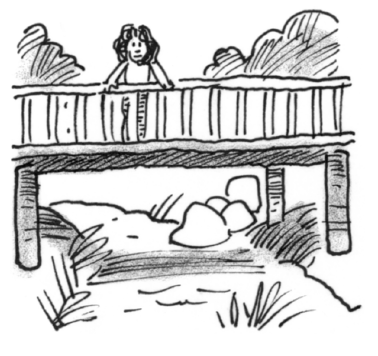### Home > CALC3RD > Chapter Ch7 > Lesson 7.3.3 > Problem7-134

7-134.Regina’s position along a straight wooden bridge is given by $x(t) = \log(t)$, where $x$ is measured in feet and t is measured in seconds. At $t = 1$ she passes her friend Angela who is going the other way, and then at $t = 10$ she stops to gaze at the water below. Homework Help ✎

1. Calculate Regina’s average velocity over the interval $1 ≤ t ≤ 10$.

$\text{average velocity}=\frac{\text{displacement}}{\text{time}}$

2. At what time in this interval was Regina traveling at her average velocity?

This is an application of the Mean Value Theorem, which guarantees that, on a closed interval, IROC = AROC somewhere if the function is continuous and differentiable.